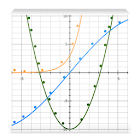Curve Fitting Tool Free

All Android applications categories

All Android games categories# Curve Fitting Tool Free

92 7.8

7.8 Users
rating

## Screenshots

Description

Scientific tool to fit given data to various non-linear equations. The app can used for curve fitting equations upto seventh degree polynomials as well as exponential, power and logarithm functions. The app is very useful for engineers, scientists, economists to analyze the non-linear system encountered in practical problems. The app has a very rich and is very user-friendly UI (user interface). The results can be sent to any e-mail id by using “Send e-mail” option in main menu.

(for curve fitting more that 10 points.Please the buy paid version which curve fit upto 50 points)

Key Features:
- Plots the original data and curve-fit equation in form of X-Y curves
-Computes “R2’ to estimates the how well the curve fits
-The percentage error of curve-fit at all points can be visualized in form of bar chart
-Can be used to interpolate and extrapolate data using the curve-fit

Application areas: Some of applications areas are:
• Physics and sciences
• Engineering
• Finance and Economics
• Industry
• Research

-Researchers
-Engineers
-Engineering students
-Science students

- Fits non-linear equation in quick time
- Reduces time in calculations.
-Reduces effort and time in research.

Have Suggestions or Issues?
Email: ashermoiblesolutions@gmail.com and you'll get a prompt response.

from 92 reviews

"Great"

7.8